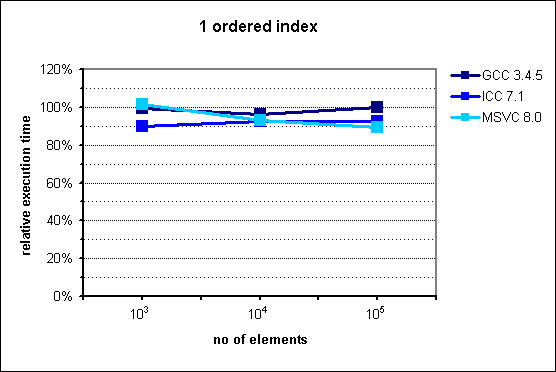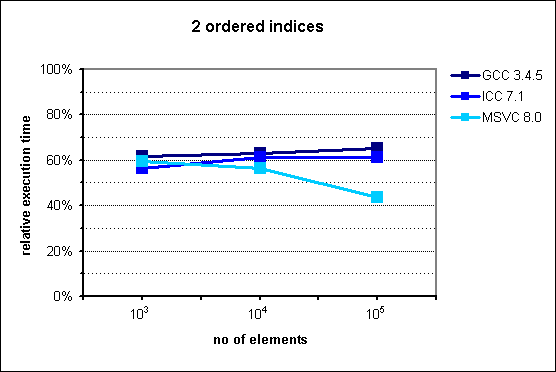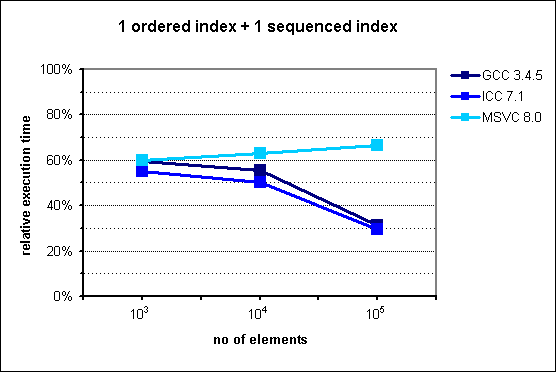#Boost C++ Libraries

...one of the most highly regarded and expertly designed C++ library projects in the world.

# Boost.MultiIndex Performance

## Introduction

Boost.MultiIndex helps the programmer to avoid the manual construction of cumbersome compositions of containers when multi-indexing capabilities are needed. Furthermore, it does so in an efficient manner, both in terms of space and time consumption. The space savings stem from the compact representation of the underlying data structures, requiring a single node per element. As for time efficiency, Boost.MultiIndex intensively uses metaprogramming techniques producing very tight implementations of member functions which take care of the elementary operations for each index: for `multi_index_container`s with two or more indices, the running time can be reduced to half as long as with manual simulations involving several STL containers.

## Manual simulation of a `multi_index_container`

The section on emulation of standard containers with `multi_index_container` shows the equivalence between single-index `multi_index_container`s and some STL containers. Let us now concentrate on the problem of simulating a `multi_index_container` with two or more indices with a suitable combination of standard containers.

Consider the following instantiation of `multi_index_container`:

```typedef multi_index_container<
int,
indexed_by<
ordered_unique<identity<int> >,
ordered_non_unique<identity<int>, std::greater >,
>
> indexed_t;
```

`indexed_t` maintains two internal indices on elements of type `int`. In order to simulate this data structure resorting only to standard STL containers, one can use on a first approach the following types:

```// dereferencing compare predicate
template<typename Iterator,typename Compare>
struct it_compare
{
bool operator()(const Iterator& x,const Iterator& y)const
{
return comp(*x,*y);
}

private:
Compare comp;
};

typedef std::set<int>  manual_t1; // equivalent to indexed_t's index #0
typedef std::multiset<
const int*,
it_compare<
const int*,
std::greater<int>
>
>                      manual_t2; // equivalent to indexed_t's index #1
```

where `manual_t1` is the "base" container that holds the actual elements, and `manual_t2` stores pointers to elements of `manual_t1`. This scheme turns out to be quite inefficient, though: while insertion into the data structure is simple enough:

```manual_t1 c1;
manual_t2 c2;

// insert the element 5
manual_t1::iterator it1=c1.insert(5).first;
c2.insert(&*it1);
```
deletion, on the other hand, necessitates a logarithmic search, whereas `indexed_t` deletes in constant time:
```// remove the element pointed to by it2
manual_t2::iterator it2=...;
c1.erase(**it2); // watch out! performs in logarithmic time
c2.erase(it2);
```

The right approach consists of feeding the second container not with raw pointers, but with elements of type `manual_t1::iterator`:

```typedef std::set<int>    manual_t1; // equivalent to indexed_t's index #0
typedef std::multiset<
manual_t1::iterator,
it_compare<
manual_t1::iterator,
std::greater<int>
>
>                        manual_t2; // equivalent to indexed_t's index #1
```

Now, insertion and deletion can be performed with complexity bounds equivalent to those of `indexed_t`:

```manual_t1 c1;
manual_t2 c2;

// insert the element 5
manual_t1::iterator it1=c1.insert(5).first;
c2.insert(it1);

// remove the element pointed to by it2
manual_t2::iterator it2=...;
c1.erase(*it2); // OK: constant time
c2.erase(it2);
```

The construction can be extended in a straightworward manner to handle more than two indices. In what follows, we will compare instantiations of `multi_index_container` against this sort of manual simulations.

## Spatial efficiency

The gain in space consumption of `multi_index_container` with respect to its manual simulations is amenable to a very simple theoretical analysis. For simplicity, we will ignore alignment issues (which in general play in favor of `multi_index_container`.)

Nodes of a `multi_index_container` with N indices hold the value of the element plus N headers containing linking information for each index. Thus the node size is

SI = e + h0 + ··· + hN-1, where
e = size of the element,
hi = size of the i-th header.

On the other hand, the manual simulation allocates N nodes per element, the first holding the elements themselves and the rest storing iterators to the "base" container. In practice, an iterator merely holds a raw pointer to the node it is associated to, so its size is independent of the type of the elements. Summing all contributions, the space allocated per element in a manual simulation is

SM = (e + h0) + (p + h1) + ··· + (p + hN-1) = SI + (N-1)p, where
p = size of a pointer.

The relative amount of memory taken up by `multi_index_container` with respect to its manual simulation is just SI / SM, which can be expressed then as:

SI / SM = SI / (SI + (N-1)p).

The formula shows that `multi_index_container` is more efficient with regard to memory consumption as the number of indices grow. An implicit assumption has been made that headers of `multi_index_container` index nodes are the same size that their analogues in STL containers; but there is a particular case in which this is often not the case: ordered indices use a spatial optimization technique which is not present in many implementations of `std::set`, giving an additional advantage to `multi_index_container`s of one system word per ordered index. Taking this fact into account, the former formula can be adjusted to:

SI / SM = SI / (SI + (N-1)p + Ow),

where O is the number of ordered indices of the container, and w is the system word size (typically 4 bytes on 32-bit architectures.)

These considerations have overlooked an aspect of the greatest practical importance: the fact that `multi_index_container` allocates a single node per element, compared to the many nodes of different sizes built by manual simulations, diminishes memory fragmentation, which can show up in more usable memory available and better performance.

## Time efficiency

From the point of view of computational complexity (i.e. big-O characterization), `multi_index_container` and its corresponding manual simulations are equivalent: inserting an element into a `multi_index_container` reduces to a simple combination of elementary insertion operations on each of the indices, and similarly for deletion. Hence, the most we can expect is a reduction (or increase) of execution time by a roughly constant factor. As we will see later, the reduction can be very significative for `multi_index_container`s with two or more indices.

In the special case of `multi_index_container`s with only one index, resulting performance will roughly match that of the STL equivalent containers: tests show that there is at most a negligible degradation with respect to STL, and even in some cases a small improvement.

## Performance tests

See source code used for measurements.

In order to assess the efficiency of `multi_index_container`, the following basic algorithm

```multi_index_container<...> c;
for(int i=0;i<n;++i)c.insert(i);
for(iterator it=c.begin();it!=c.end();)c.erase(it++);
```

has been measured for different instantiations of `multi_index_container` at values of n 1,000, 10,000 and 100,000, and its execution time compared with that of the equivalent algorithm for the corresponding manual simulation of the data structure based on STL containers. The table below describes the test environments used.

Tests environments.
Compiler Settings OS and CPU
GCC 3.4.5 (mingw special) `-O3` Windows 2000 Pro on P4 1.5 GHz, 256 MB RAM
Intel C++ 7.1 default release settings Windows 2000 Pro on P4 1.5 GHz, 256 MB RAM
Microsoft Visual C++ 8.0 default release settings, `_SECURE_SCL=0` Windows XP on P4 Xeon 3.2 GHz, 1 GB RAM

The relative memory consumption (i.e. the amount of memory allocated by a `multi_index_container` with respect to its manual simulation) is determined by dividing the size of a `multi_index_container` node by the sum of node sizes of all the containers integrating the simulating data structure.

### Results for 1 ordered index

The following instantiation of `multi_index_container` was tested:

```multi_index_container<
int,
indexed_by<
ordered_unique<identity<int> >
>
>
```

which is functionally equivalent to `std::set<int>`.

#### Memory consumption

GCC 3.4.5 ICC 7.1 MSVC 8.0
80% 80% 80%
Table 1: Relative memory consumption of `multi_index_container` with 1 ordered index.

The reduction in memory usage is accounted for by the optimization technique implemented in Boost.MultiIndex ordered indices, as explained above.

#### Execution timeFig. 1: Performance of `multi_index_container` with 1 ordered index.

Somewhat surprisingly, `multi_index_container` performs slightly better than `std::set`. A very likely explanation for this behavior is that the lower memory consumption of `multi_index_container` results in a higher processor cache hit rate. The improvement is smallest for GCC, presumably because the worse quality of this compiler's optimizer masks the cache-related benefits.

### Results for 1 sequenced index

The following instantiation of `multi_index_container` was tested:

```multi_index_container<
int,
indexed_by<
sequenced<>
>
>
```

which is functionally equivalent to `std::list<int>`.

#### Memory consumption

GCC 3.4.5 ICC 7.1 MSVC 8.0
100% 100% 100%
Table 2: Relative memory consumption of `multi_index_container` with 1 sequenced index.

The figures confirm that in this case `multi_index_container` nodes are the same size than those of its `std::list` counterpart.

#### Execution timeFig. 2: Performance of `multi_index_container` with 1 sequenced index.

`multi_index_container` does not attain the performance of its STL counterpart, although the figures are close. Again, the worst results are those of GCC, with a degradation of up to 7%, while ICC and MSVC do not exceed a mere 5%.

### Results for 2 ordered indices

The following instantiation of `multi_index_container` was tested:

```multi_index_container<
int,
indexed_by<
ordered_unique<identity<int> >,
ordered_non_unique<identity<int> >
>
>
```

#### Memory consumption

GCC 3.4.5 ICC 7.1 MSVC 8.0
70% 70% 70%
Table 3: Relative memory consumption of `multi_index_container` with 2 ordered indices.

These results concinde with the theoretical formula for SI = 28, N = O = 2 and p = w = 4.

#### Execution timeFig. 3: Performance of `multi_index_container` with 2 ordered indices.

The experimental results confirm our hypothesis that `multi_index_container` provides an improvement on execution time by an approximately constant factor, which in this case lies around 60%. There is no obvious explanation for the increased advantage of `multi_index_container` in MSVC for n=105.

### Results for 1 ordered index + 1 sequenced index

The following instantiation of `multi_index_container` was tested:

```multi_index_container<
int,
indexed_by<
ordered_unique<identity<int> >,
sequenced<>
>
>
```

#### Memory consumption

GCC 3.4.5 ICC 7.1 MSVC 8.0
75% 75% 75%
Table 4: Relative memory consumption of `multi_index_container` with 1 ordered index + 1 sequenced index.

These results concinde with the theoretical formula for SI = 24, N = 2, O = 1 and p = w = 4.

#### Execution timeFig. 4: Performance of `multi_index_container` with 1 ordered index + 1 sequenced index.

For n=103 and n=104, the results are in agreement with our theoretical analysis, showing a constant factor improvement of 50-65% with respect to the STL-based manual simulation. Curiously enough, this speedup gets even higher when n=105 for two of the compilers, namely GCC and ICC. In order to rule out spurious results, the tests have been run many times, yielding similar outcoumes. Both test environments are deployed on the same machine, which points to some OS-related reason for this phenomenon.

### Results for 3 ordered indices

The following instantiation of `multi_index_container` was tested:

```multi_index_container<
int,
indexed_by<
ordered_unique<identity<int> >,
ordered_non_unique<identity<int> >,
ordered_non_unique<identity<int> >
>
>
```

#### Memory consumption

GCC 3.4.5 ICC 7.1 MSVC 8.0
66.7% 66.7% 66.7%
Table 5: Relative memory consumption of `multi_index_container` with 3 ordered indices.

These results concinde with the theoretical formula for SI = 40, N = O = 3 and p = w = 4.

#### Execution timeFig. 5: Performance of `multi_index_container` with 3 ordered indices.

Execution time for this case is between 45% and 55% lower than achieved with an STL-based manual simulation of the same data structure.

### Results for 2 ordered indices + 1 sequenced index

The following instantiation of `multi_index_container` was tested:

```multi_index_container<
int,
indexed_by<
ordered_unique<identity<int> >,
ordered_non_unique<identity<int> >,
sequenced<>
>
>
```

#### Memory consumption

GCC 3.4.5 ICC 7.1 MSVC 8.0
69.2% 69.2% 69.2%
Table 6: Relative memory consumption of `multi_index_container` with 2 ordered indices + 1 sequenced index.

These results concinde with the theoretical formula for SI = 36, N = 3, O = 2 and p = w = 4.

#### Execution timeFig. 6: Performance of `multi_index_container` with 2 ordered indices + 1 sequenced index.

In accordance to the expectations, execution time is improved by a fairly constant factor, which ranges from 45% to 55%.

## Conclusions

We have shown that `multi_index_container` outperforms, both in space and time efficiency, equivalent data structures obtained from the manual combination of STL containers. This improvement gets larger when the number of indices increase.

In the special case of replacing standard containers with single-indexed `multi_index_container`s, the performance of Boost.MultiIndex is comparable with that of the tested STL implementations, and can even yield some improvements both in space consumption and execution time.

Revised May 9th 2006